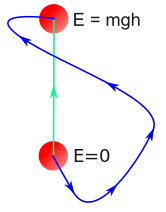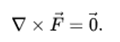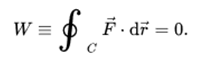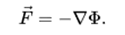Courses

# Conservative Forces Mechanical Engineering Notes | EduRev

## Mechanical Engineering : Conservative Forces Mechanical Engineering Notes | EduRev

The document Conservative Forces Mechanical Engineering Notes | EduRev is a part of the Mechanical Engineering Course Engineering Mechanics - Notes, Videos, MCQs & PPTs.
All you need of Mechanical Engineering at this link: Mechanical Engineering

A conservative force is a force with the property that the total Work done in moving a particle between two points is independent of the taken path. Equivalently, if a particle travels in a closed loop, the net work done (the sum of the force acting along the path multiplied by the displacement) by a conservative force is zero.

A conservative force is dependent only on the position of the object. If a force is conservative, it is possible to assign a numerical value for the potential at any point. When an object moves from one location to another, the force changes the potential energy of the object by an amount that does not depend on the path taken. If the force is not conservative, then defining a scalar potential is not possible, because taking different paths would lead to conflicting potential differences between the start and end points.

Gravitational force is an example of a conservative force, while frictional forceis an example of a non-conservative force.Other examples of conservative forces are: force in elastic spring, electrostatic force between two electric charges, magnetic force between two magnetic poles. The last two forces are called central forces as they act along the line joining the centres of two charged/magnetized bodies. Thus, all central forces are conservative forces.

Informal definition

Informally, a conservative force can be thought of as a force that conserves mechanical energy. Suppose a particle starts at point A, and there is a force F acting on it. Then the particle is moved around by other forces, and eventually ends up at A again. Though the particle may still be moving, at that instant when it passes point A again, it has traveled a closed path. If the net work done by F at this point is 0, then F passes the closed path test. Any force that passes the closed path test for all possible closed paths is classified as a conservative force.

The gravitational force, spring force, magnetic force (according to some definitions, see below) and electric force (at least in a time-independent magnetic field, see Faraday's law of inductionfor details) are examples of conservative forces, while friction and air drag are classical examples of non-conservative forces.

For non-conservative forces, the mechanical energy that is lost (not conserved) has to go somewhere else, by conservation of energy. Usually the energy is turned into heat, for example the heat generated by friction. In addition to heat, friction also often produces some sound energy. The water drag on a moving boat converts the boat's mechanical energy into not only heat and sound energy, but also wave energy at the edges of its wake. These and other energy losses are irreversible because of the second law of thermodynamics.

Path independence

A direct consequence of the closed path test is that the work done by a conservative force on a particle moving between any two points does not depend on the path taken by the particle.

This is illustrated in the figure to the right: The work done by the gravitational force on an object depends only on its change in height because the gravitational force is conservative. The work done by a conservative force is equal to the negative of change in potential energy during that process. For a proof, imagine two paths 1 and 2, both going from point A to point B. The variation of energy for the particle, taking path 1 from A to B and then path 2 backwards from B to A, is 0; thus, the work is the same in path 1 and 2, i.e., the work is independent of the path followed, as long as it goes from A to B.

For example, if a child slides up a frictionless slide, the work done by the gravitational force on the child from the down of the slide to the up will be the same no matter what the shape of the slide; it can be straight or it can be a spiral or conical. The amount of work done only depends on the vertical displacement of the childMathematical description

A force field F, defined everywhere in space (or within a simply-connected volume of space), is called a conservative force or conservative vector field if it meets any of these three equivalent conditions:

1. The curl of F is the zero vector:2. There is zero net Work (W) done by the force when moving a particle through a trajectory that starts and ends in the same place:3. The force can be written as the negative gradient of a potential,

##The term conservative force comes from the fact that when a conservative force exists, it conserves mechanical energy. The most familiar conservative forces are gravity, the electric force (in a time-independent magnetic field, see Faraday's law), and spring force.

Offer running on EduRev: Apply code STAYHOME200 to get INR 200 off on our premium plan EduRev Infinity!

,

,

,

,

,

,

,

,

,

,

,

,

,

,

,

,

,

,

,

,

,

;